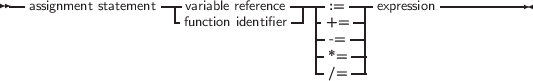#### 13.1.1 Assignments

Assignments give a value to a variable, replacing any previous value the variable might have had:

_________________________________________________________________________________________________________
Assignments___________________________________________________________________

In addition to the standard Pascal assignment operator (:=), which simply replaces the value of the variable with the value resulting from the expression on the right of the := operator, Free Pascal supports some C-style constructions. All available constructs are listed in table (13.1).

Table 13.1: Allowed C constructs in Free Pascal

 Assignment Result a += b Adds b to a, and stores the result in a. a -= b Subtracts b from a, and stores the result in a. a *= b Multiplies a with b, and stores the result in a. a /= b Divides a through b, and stores the result in a.

For these constructs to work, the -Sc command-line switch must be specified.

Remark These constructions are just for typing convenience, they don’t generate different code. Here are some examples of valid assignment statements:

X := X+Y;
X+=Y;      { Same as X := X+Y, needs -Sc command line switch}
X/=2;      { Same as X := X/2, needs -Sc command line switch}
Done := False;
Weather := Good;
MyPi := 4* Tan(1);

Keeping in mind that the dereferencing of a typed pointer results in a variable of the type the pointer points to, the following are also valid assignments:

Var
L : ^Longint;
P : PPChar;

begin
L^:=3;
P^^:=’A’;

Note the double dereferencing in the second assignment.